## CodeNewbie Community is a community of 4,499 amazing newbies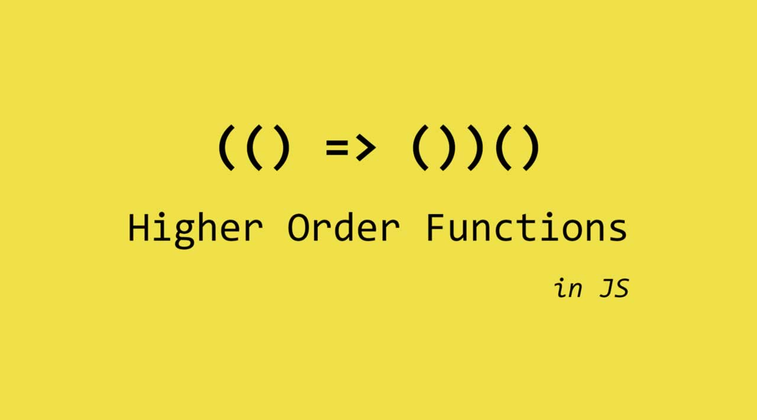# High order functions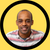Denis Woldemariam
Software Developer, Writer✍, love learning & building projects. Blockchain enthusiast! Addicted to Books 📚. I run for meditation🏃🏾‍♂️

Higher order function are functions that take in other functions as arguments or return functions. What do I am mean by that?

Let’s say we want to create a function that performs addition & another one that performs multiplication.

So f(x) => x + 3 ...................(1)

And then a function that performs multiplication.

g(x) => 4x .................................(2)

These functions perform basic arithmetic. Both take in arguments or rather inputs & give out the results as output after performing an operation.

For example: let say x = 4

If we put x in f(x), then f(4) = 7
If we put x in g(x), then g(4) = 16

That’s very simple & straight forward. These two functions are first order functions which take in variables or numbers as inputs and produce a result as output after performing an operation.

Now what if we want to put in a number in the f(x) and use the resulting output as input for g(x).

Let’s assume x = 4

f(4) => 4 + 3
f(4) => 7

Then take 7 and use it as input in g(x)

g(7) => 7 * 4
g(7) => 28

But this process is inefficient and takes times. You don’t want to waist time, energy & brain power since that’s potentially a lost revenue. Plus programmers are prone to making more mistakes the longer the code & the more complex it is. This is where higher order functions come in. Higher order functions are functions that either take in functions as arguments and/or return functions as the output.

Instead of performing the two operations using one function after another both can be performed with one function that combines the operations performed by both functions.

How does this play out?

Well the above example can be performed by using f(x) as input in g(x) and we will get the exact same result. And this is what creates a higher order function. A function that takes another function as argument and/or return function as output.

Let’s perform the above operations using higher order function

g(f(x)) => 4(x+3)
g(f(x)) => 4x + 12

g(f(x)) is a higher order function.

So g(f(4)) => 4(4) + 12

g(f(4)) => 28 which is exactly the same answer.

This shows that higher-order functions can be great when you need to compose operations. When you want to perform multiple operations in series. Higher order functions streamline the whole process & help make data processing and data manipulation easy, cost-effective & efficient. This is great considering a huge aspect of software development or tech in general is all about processing and manipulating data. Now there are several important higher order functions that are regularly used by software developers. These are :-

• forEach
• Filter
• Map
• Sort
• Reduce

It is important to learn and understand these higher order functions. Each high order function passes another function as an argument & usually take in or pass an iterator, index or the whole array.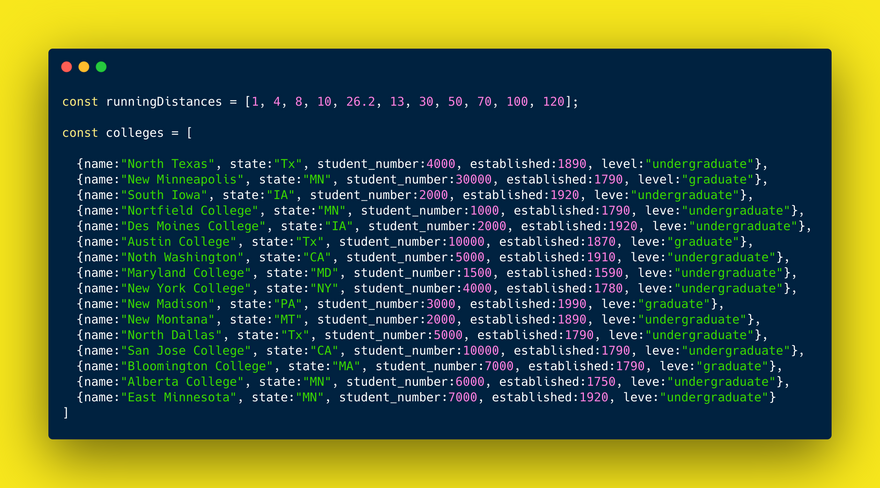• forEach is a method or function used to loop through data in array in a better or efficient way as suppose to a for loop. It does not in anyway return a new set of arrays. I.e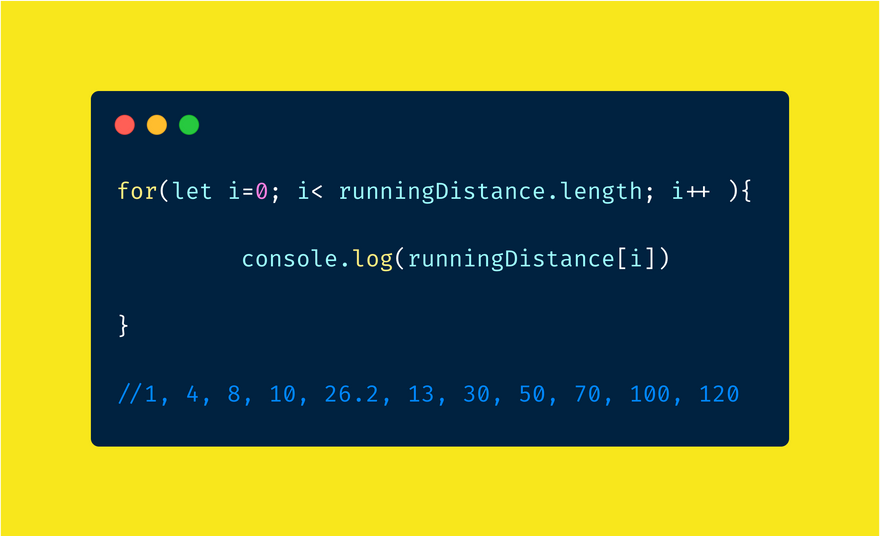Let’s see how we can get the same result with forEach in a more efficient or elegant way with less code.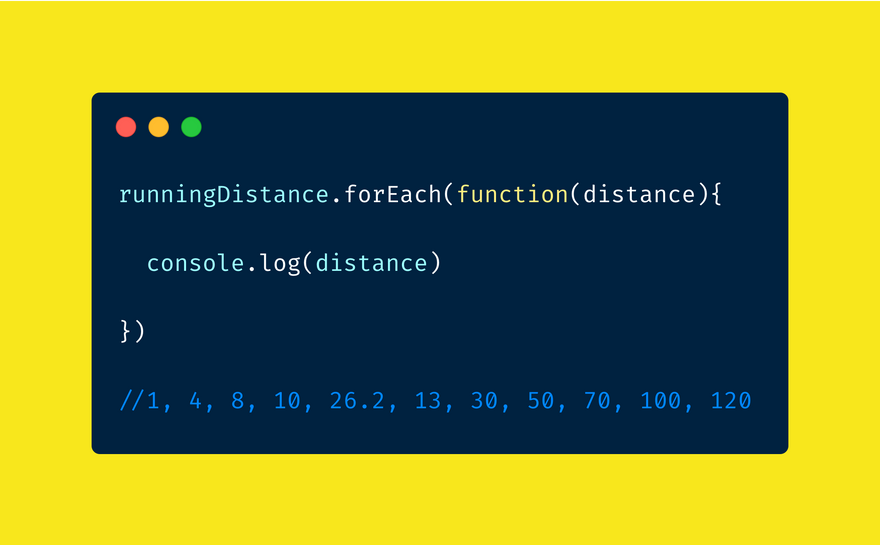Both give out the same result.

• Filter is a higher order function used to filter out data. It tests the data with the criteria you have set and return those sets of data that passed the test or are true.

Let's look how we can filter using for loop and if statements.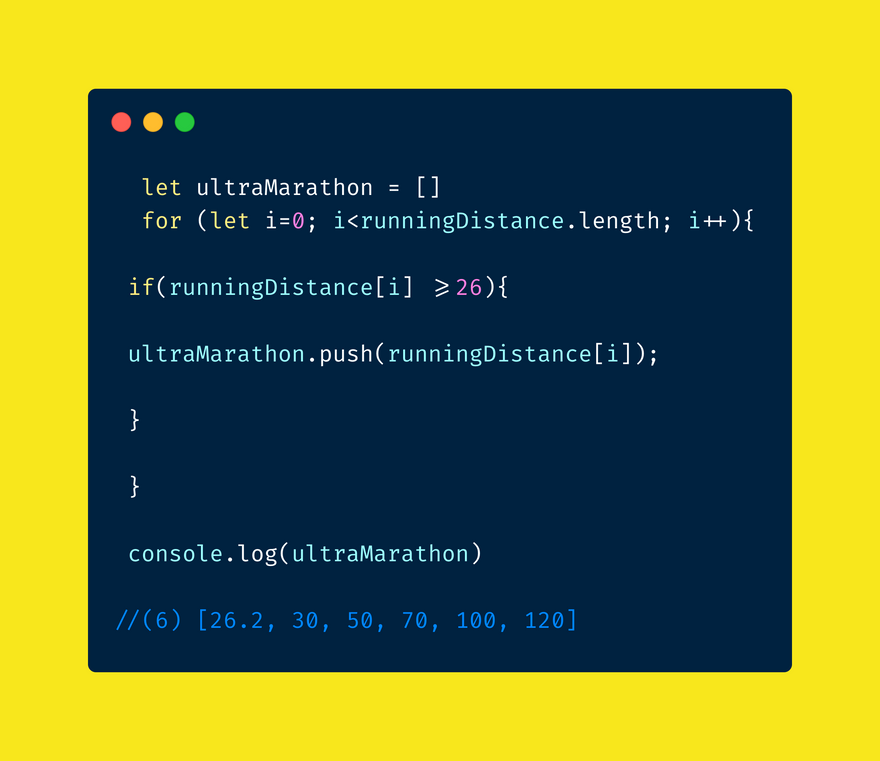Now let's look how we can accomplish the same thing with filter.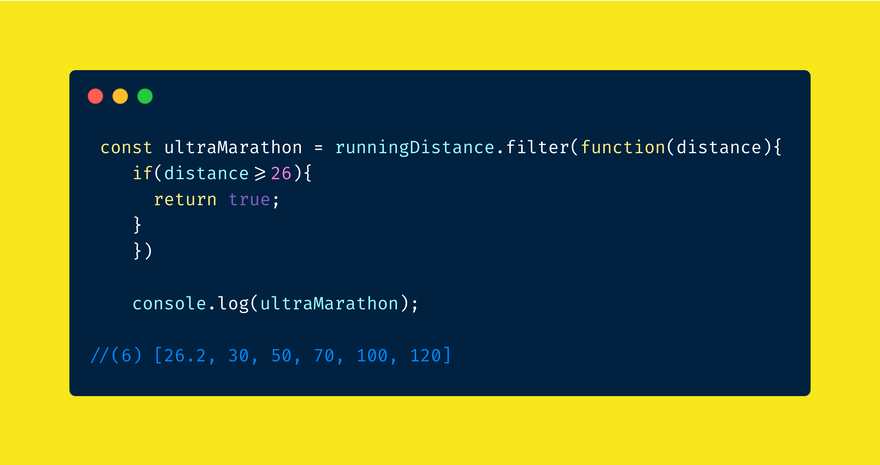We can even make it even shorter & more elegant and get the same result.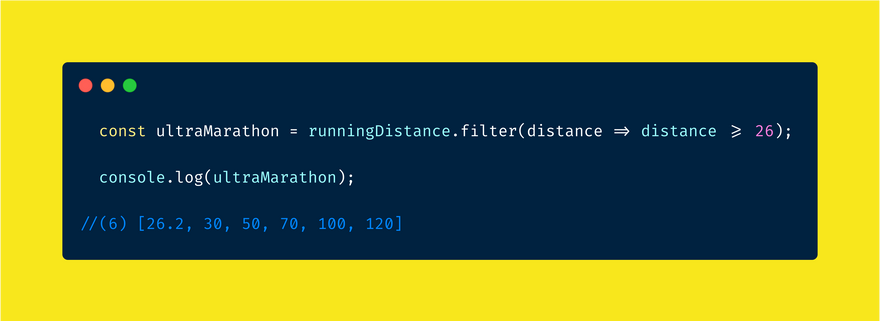Here is another example:-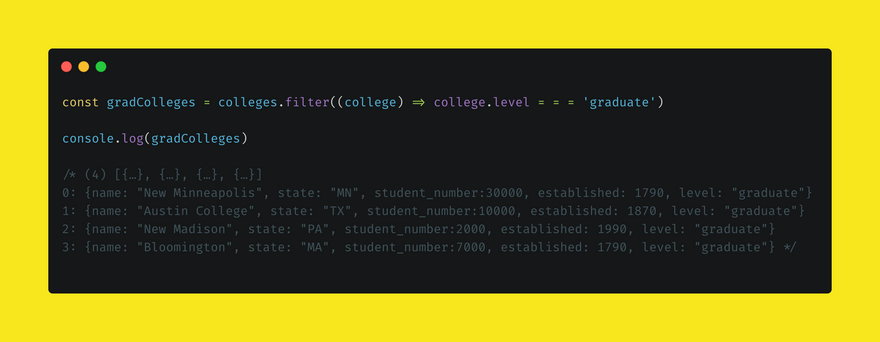• Map is a higher order function you can use in which you loop through the array to manipulate & transform the data and return a new set of array.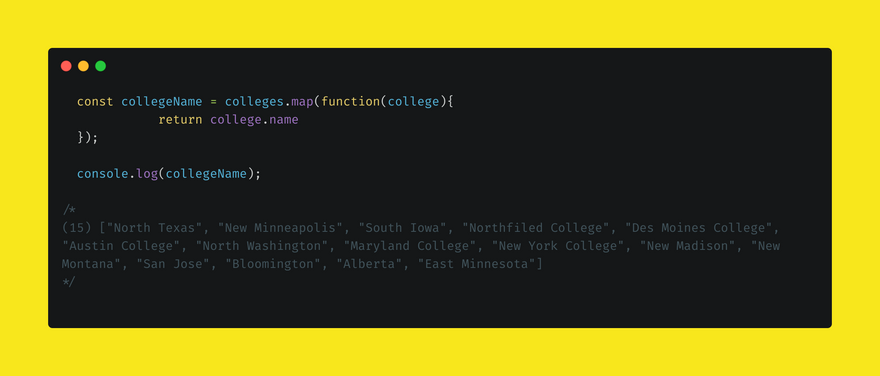Here is another example with arrow function:-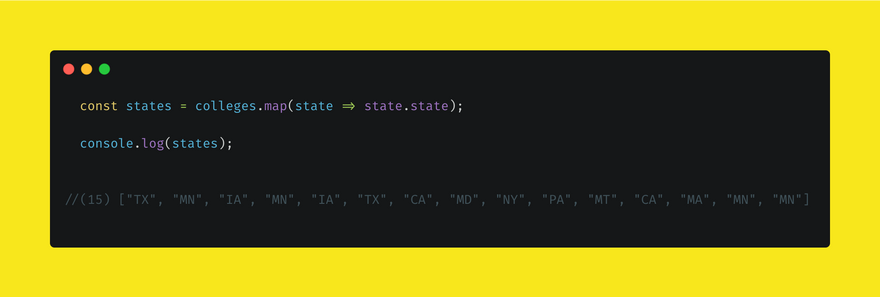• Sort is a higher order function that sorts data based on criteria we have set in ascending or descending order. It accomplishes this by taking two sets of data and comparing them based on the criteria & then arranging them in ascending or descending order.i.e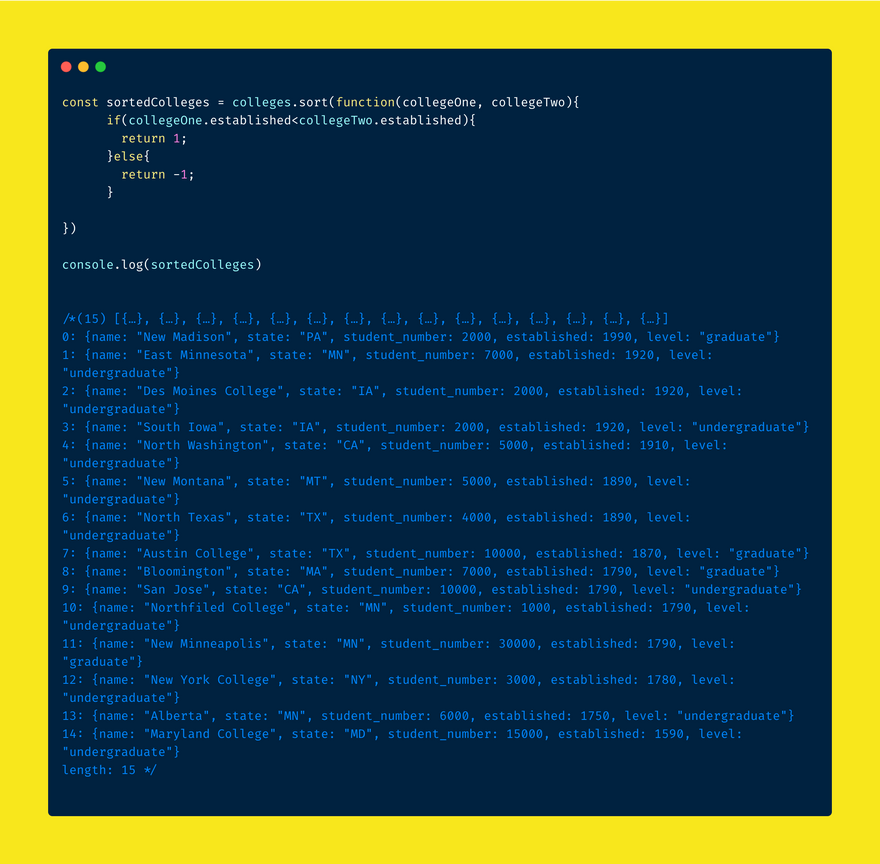As you can see above the colleges where sorted by established date in descending order.

Here is another example. This time using arrow function to sort the running distance in ascending order.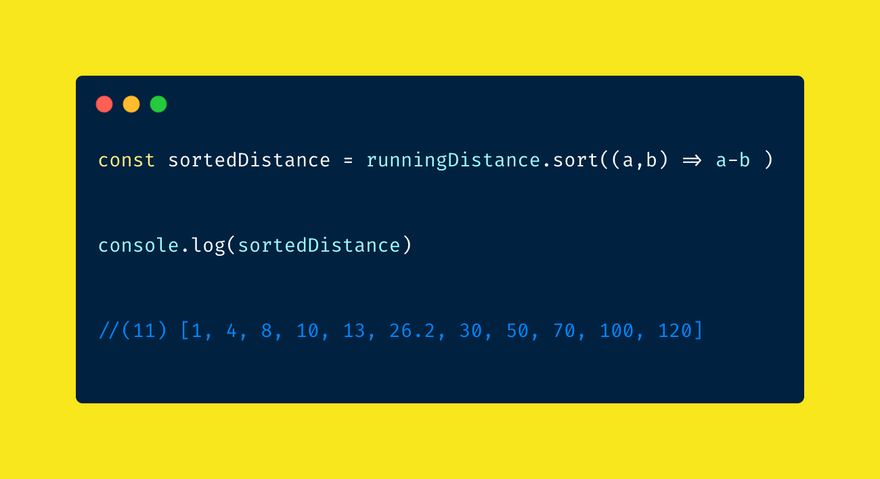• Reduce is a higher order function or method used to reduce or add the data in the array to a single total value. It passes the accumulator, current value and initial value.

let's get the total sum of the running distance using a for loop to understand how reduce works through comparison.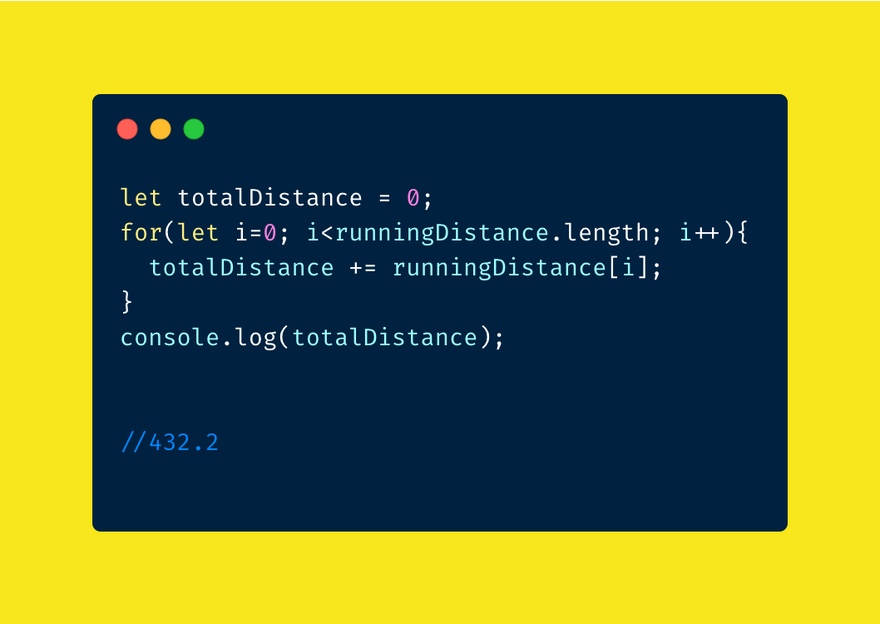The same operation can be performed using reduce in an easier and efficient way.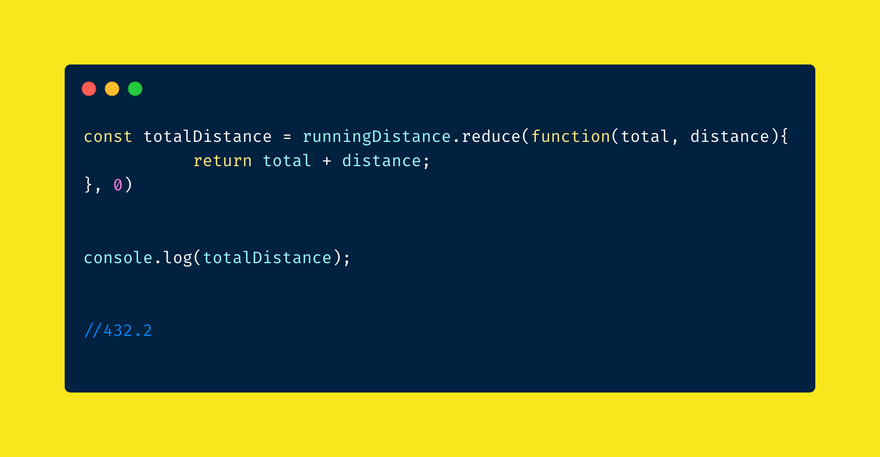This can be written using arrow function as well.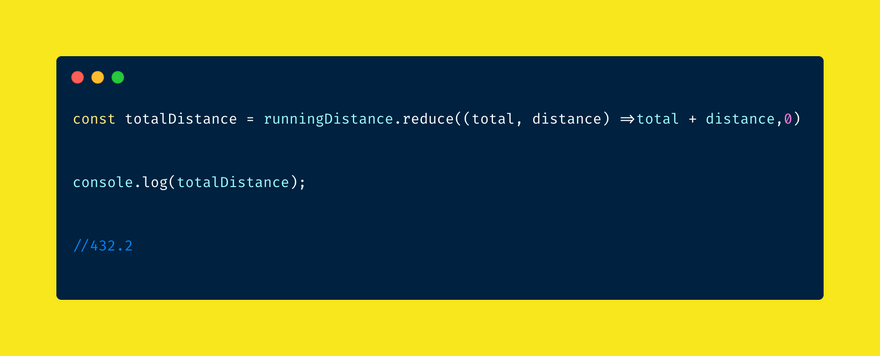In conclusion it can be beneficial to use higher order functions. Indeed there are many cases in which it is better to use higher order functions instead of using multiple functions with multiple operations to solve your problem. Higher order functions reduce the amount of code that is written & make it less complicated. This decreases the possibility of introducing bugs. By streamlining the whole process, data processing & manipulation can be made to be efficient, easier & cost effective.Therefore it is important to know & understand higher order functions really well.

I hope I did a good job of explaining this concept. Please let me know what you think and you can follow me on codnewbie or on twitter at @wolde_ai to get similar content.

## Discussion (3)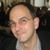Hi Denis!

This looks awesome. I think all of your examples fall into the first category (functions that take functions as arguments), but your definition ("A function that takes another function as argument and/or return function as output") highlights there's also a second kind of higher order function, that returns functions as output, one possible example is `compose`, which probably looks something like this

``````compose = (f1, f2) => (x) =>  f1( f2(x) )
``````

Then your `g(f(x))` example from the beginning is just `compose(g,f)`, and you can continue abstracting, and compose composed functions - like

``````f = (x) => x + 3
g = (x) => 4 * x
compose( compose(g,g), compose(f,g) )(4)
304
``````

Another example would be `repeat` which would take a function, and compose it on itself a number n times, like

`````` repeat = (f,n) => ((n == 1) ? f : compose(f, repeat(f, n -1)))
``````

I'm not sure if that kind of higher order function is built into javascript already?Denis Woldemariam

No I don't think so. Don't quote me though because I am still learning about it. I think you have a point. The point of the article was to introduce developers to the most used higher order functions in javaScript and that is the reason why I focused on the first kind. I should have given examples to the second as well but like you said I am not sure if we have those in javaScript. I will try to do some more research and see if I can give examples to that. Thank you for the feedbackYeah - I think I did a little looking around after I asked that question (MDN has a section on closures that's relevant), and it looks like there are performance reasons to not create functions at runtime the way I was showing - the JS runtime is able to do some optimizations when the functions are expressed in the code - and less able to do that when they're created in memory from a function call (like my `compose` example).